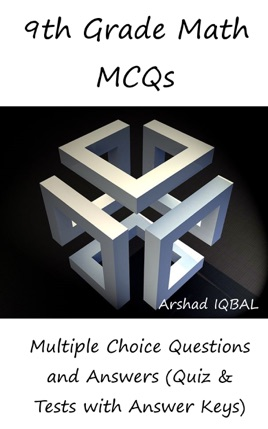• \$3.99

## Publisher Description

"9th Grade Math MCQs: Multiple Choice Questions and Answers (Quiz & Tests with Answer Keys)" provides mock tests for competitive exams preparation. "Grade 9 Math MCQ" PDF helps with fundamental concepts, analytical, and theoretical learning for self-assessment study skills. 9th Grade Math Quizzes, a quick study guide can help to learn and practice questions for placement test preparation.
"Grade 9 Math Multiple Choice Questions and Answers (MCQs)" PDF to download is a revision guide with a collection of trivia questions to fun quiz questions and answers PDF on topics: Algebraic expressions and algebraic formulas, algebraic manipulation, arithmetic and geometric sequences, basic math problems, basic statistics, business mathematics, congruent triangles and geometry, consumer math, factorization, introduction to logarithms, linear equations and inequalities, linear graphs and applications, logarithms and exponents, mathematical theorems, matrices and determinants, percentage, ratio and proportion, real and complex numbers, sets and functions for learning.
"Grade 9 Math Questions and Answers" PDF covers certification exam syllabus, competitive exam papers with answers, and career tests prep from math textbooks on chapters:
Algebraic Expressions and Algebraic Formulas MCQs: 49 Multiple Choice Questions. Algebraic Manipulation MCQs: 13 Multiple Choice Questions. Arithmetic and Geometric Sequences MCQs: 36 Multiple Choice Questions. Basic Math Problems MCQs: 20 Multiple Choice Questions. Basic Statistics MCQs: 35 Multiple Choice Questions. Business Mathematics MCQs: 37 Multiple Choice Questions. Congruent Triangles and Geometry MCQs: 17 Multiple Choice Questions. Consumer Math MCQs: 20 Multiple Choice Questions. Factorization MCQs: 13 Multiple Choice Questions. Introduction to Logarithms MCQs: 30 Multiple Choice Questions. Linear Equations and Inequalities MCQs: 27 Multiple Choice Questions. Linear Graphs and Applications MCQs: 16 Multiple Choice Questions. Logarithms and Exponent.s MCQs: 12 Multiple Choice Questions. Mathematical Theorems MCQs: 24 Multiple Choice Questions. Matrices and Determinants MCQs: 48 Multiple Choice Questions. Percentage, Ratio and Proportion MCQs: 51 Multiple Choice Questions. Real and Complex Numbers MCQs: 61 Multiple Choice Questions. Sets and Functions MCQs: 30 Multiple Choice Questions.
"Algebraic Expressions and Algebraic Formulas MCQ" PDF covers quiz questions about algebraic expressions, algebra formulas, surds, rationalization of surds, and applications.
"Algebraic Manipulation MCQ" PDF covers quiz questions about square root of algebraic expression, basic mathematics, LCM, and HCF.
"Arithmetic and Geometric Sequences MCQ" PDF covers quiz questions about arithmetic sequence, arithmetic mean, geometric sequence, and geometric mean.
"Basic Math Problems MCQ" PDF covers quiz questions about math theorems, collinear points, distance formula, mid-point formula, Pythagoras theorem, and solving linear inequalities.
"Basic Statistics MCQ" PDF covers quiz questions about central tendency measurements, central tendency: mean, median and mode, measures of central tendency, cumulative frequency, frequency distribution, and measures of dispersion.